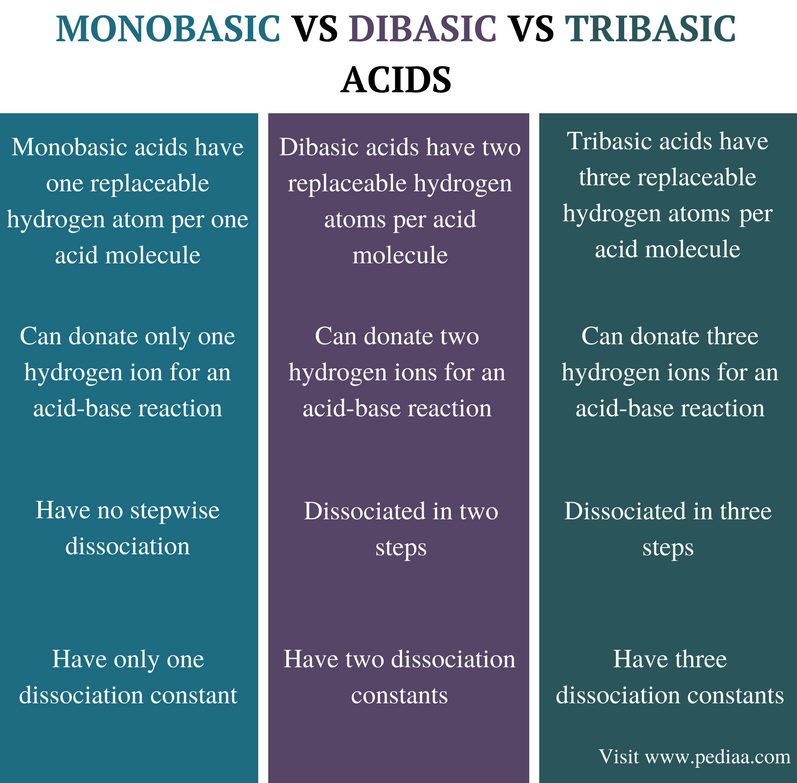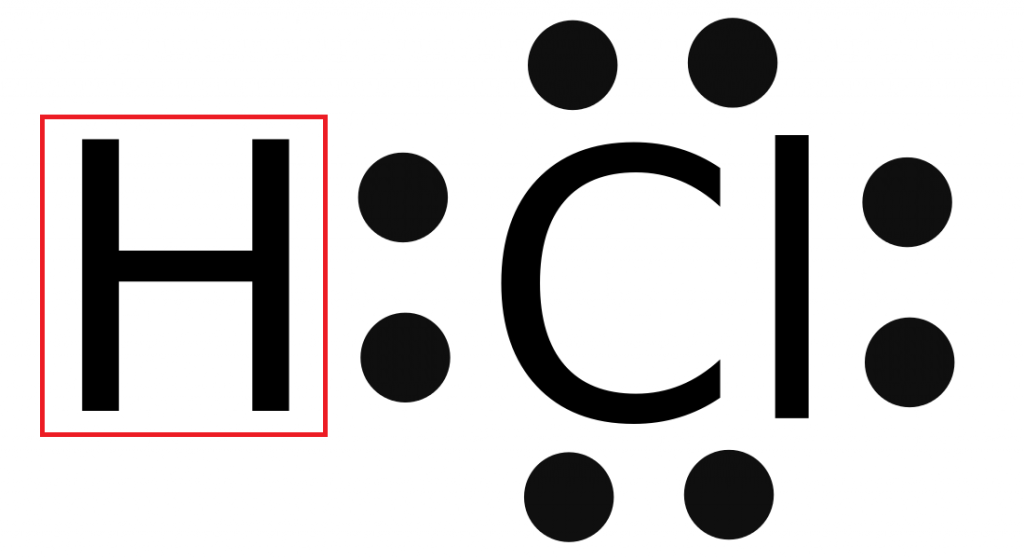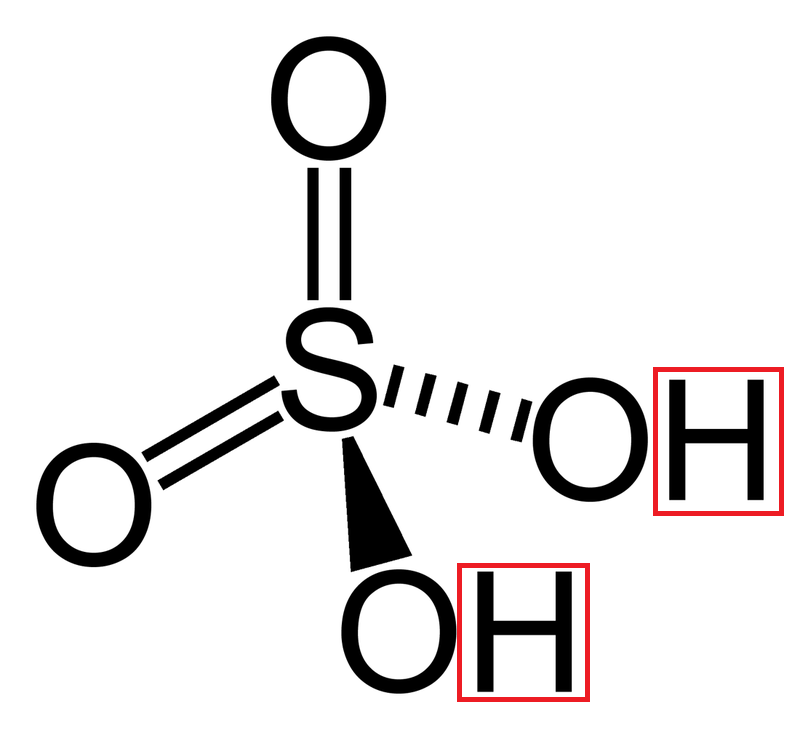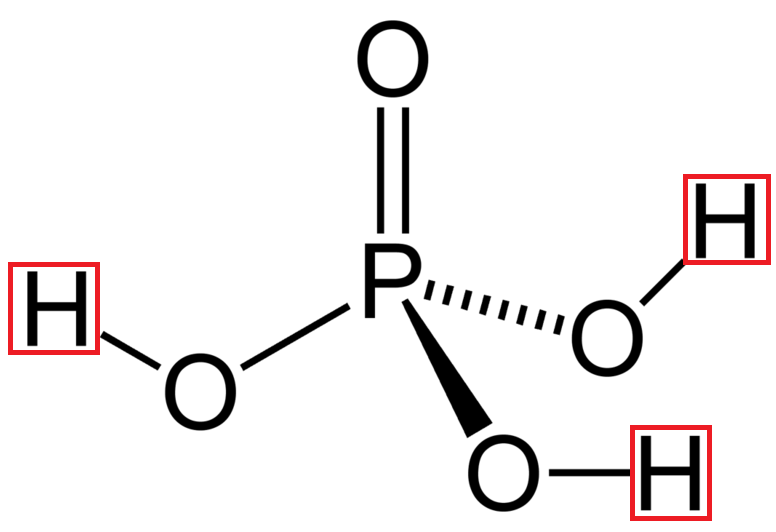# Difference Between Monobasic Dibasic and Tribasic Acids

## Main Difference – Monobasic vs Dibasic vs Tribasic Acids

Acids are chemical compounds that have acidic properties. An acid can also be defined as a chemical species that can react with a base forming a salt and water. There are two main types of acids as strong acids and weak acids. Acids can also be categorized into three groups as monobasic acids, dibasic acids, and tribasic acids. Acids are grouped in such a way according to the number of protons they have in order to react with a base. Dibasic and tribasic acids together are called polybasic acids. These monobasic and polybasic acids can be either strong acids or weak acids. The main difference between monobasic dibasic and tribasic acids is that a monobasic acid has only one replaceable hydrogen atom and a dibasic acid has two replaceable hydrogen atoms whereas a tribasic acid has three replaceable hydrogen atoms.

### Key Areas Covered

1. What are Monobasic Acids

– Definition, Dissociation, and Examples
2. What are Dibasic Acids

– Definition, Dissociation, and Examples
3. What are Tribasic Acids

– Definition, Dissociation, and Examples
4. What is the Difference Between Monobasic Dibasic and Tribasic Acids

– Comparison of Key Differences

Key Terms: Acid, Dibasic Acids, Monobasic Acid, Salt, Tribasic Acid## What are Monobasic Acids

Monobasic acids are acidic compounds that have one replaceable hydrogen atom per one acid molecule. In an aqueous solution, these acids can remove one proton (a hydrogen atom). Therefore, these are called monoprotic acids. A monobasic acid can donate only one hydrogen atom for the reaction between a monobasic acid and a base.

Since the dissociation of a monobasic acid donates only one hydrogen atom to the system, the equation for the dissociation constant of a monobasic acid includes the concentration of only one H+ ion. The dissociation of a monobasic acid can be shown as below.

HA(aq)  →  H+(aq)    +   A(aq)

or

HA(aq)    +  H2O(l)     →   A(aq) +   H3O+(aq)

Dissociation constant Ka  =  [H+(aq)][ A(aq)]  / [HA(aq)]Figure 1: Structure of Hydrochloric Acid ( The Hydrogen atom is shown in red)

There are organic and inorganic monobasic acids. Some are strong acids whereas others are weak acids. Strong monobasic acids such as HCl, HNO3, HBr can completely dissociate and donate one hydrogen to a base. Weak monobasic acids such as acetic acid (CH3COOH) are partially dissociated.

## What are Dibasic Acids

Dibasic acids are chemical compounds that have two replaceable hydrogen atoms per acid molecule. Therefore these dibasic acids can donate two hydrogen atoms for the reaction between a dibasic acid and a base. In an aqueous solution, dibasic acids dissociate providing two hydrogen ions (H+) to the system. Therefore, these are also called diprotic acids.

The dissociation of a dibasic acid can be given as below. A dibasic acid has two dissociation constant values. These are for the first dissociation and the second dissociation.

H2B(aq)  →   2H+(aq)   +    B-2(aq)

or

H2B(aq)   →   H+(aq)    +    HB(aq) ; Ka1

HB(aq)   →    H+(aq)    +    B-2(aq)  ; Ka2

Dissociation constant Ka1   =    [H+(aq)][ HB(aq)]  /  [H2B(aq)]

Dissociation constant Ka2   =    [H+(aq)][ B-2(aq)]  /  [HB(aq)]Figure 2: Structure of Sulfuric Acid ( Two Hydrogen atoms are shown in red)

Dibasic acids can also be found as two types as strong dibasic acids and weak dibasic acids. Strong dibasic acids completely dissociate into two hydrogen atoms and the conjugated ion of the acid. Their first ionization is strong. But weak dibasic acids partially dissociate into two hydrogen atoms and the conjugated ion of the acid. Some common examples of dibasic acids include H2SO4, H2CO3 and H2C2O4

## What are Tribasic Acids

Tribasic acids are chemical compounds that have three replaceable hydrogen atoms. These acids can donate three hydrogen ions for the reaction between a tribasic acid and a base. Since they are able to remove three H+ ions (protons), these acids are also called triprotic acids.

The dissociation of a tribasic acid provides three hydrogen ions to the system. Therefore, the dissociation of a tribasic acid has three dissociation constants.

H3C(aq)    →     3H+(aq)   +    C-3(aq)

or

H3C(aq)    →  H+(aq)   +  H2C(aq)   ; Ka1

H2C(aq)    →     H+(aq)     +    HC-2(aq)      ; Ka2

HC-2(aq)    →      H+(aq)     +    C-3(aq)     ; Ka3

Dissociation constant, Ka1   =    [H+(aq)][ H2C(aq)]  /  [H3C(aq)]

Dissociation constant, Ka2   =    [H+(aq)][ HC-2(aq)]  /  [H2C(aq)]

Dissociation constant, Ka3   =    [H+(aq)][ C-3(aq)]  /  [HC-2(aq)]Figure 3: Structure of Phosphoric Acid (Three Hydrogen atoms are shown in red)

A common inorganic tribasic acid is phosphoric acid (H3PO4). It is composed of three hydrogen atoms bonded to three oxygen atoms around the phosphorous atom. These hydrogen atoms can be replaced or removed from the molecule. A common organic tribasic acid is citric acid

## Difference Between Monobasic Dibasic and Tribasic Acids

### Definition

Monobasic Acid: Monobasic acids are acidic compounds that have one replaceable hydrogen atom per acid molecule.

Dibasic Acid: Dibasic acids are chemical compounds that have two replaceable hydrogen atoms per acid molecule.

Tribasic Acid: Tribasic acids are chemical compounds that have three replaceable hydrogen atoms per acid molecule.

### Hydrogen Ions Donated for Acid-Base Reactions

Monobasic Acid: Monobasic acids can donate only one hydrogen ion for an acid-base reaction.

Dibasic Acid: Dibasic acids can donate two hydrogen ions for an acid-base reaction.

Tribasic Acid: Tribasic acids can donate three hydrogen ions for an acid-base reaction.

### Dissociation

Monobasic Acid: Monobasic acids have no stepwise dissociation.

Dibasic Acid: Dibasic acids are dissociated in two steps.

Tribasic Acid: Tribasic acids are dissociated in three steps.

### Dissociation Constants

Monobasic Acid: Monobasic acids have only one dissociation constant.

Dibasic Acid: Dibasic acids have two dissociation constants.

Tribasic Acid: Tribasic acids have three dissociation constants.

### Conclusion

Acids are compounds that can release protons (or H+ ions) to a system. Therefore, the presence of an acid is indicated by a low pH value of that system. The acidity of a system is dependent on the type of acid present in that system. Acids are mainly categorized as strong acids and weak acids. According to the number of protons that these acids donate for an acid-base reaction, acids can also be grouped as monobasic acids and polybasic acids. Dibasic acids and tribasic acids are polybasic acids. The main difference between monobasic dibasic and tribasic acids is that a monobasic acid has only one replaceable hydrogen atom and dibasic acids have two replaceable hydrogens whereas tribasic acids have three replaceable hydrogen atoms.

##### References:

1. “Acid.” Wikipedia, Wikimedia Foundation, 23 Aug. 2017, Available here. Accessed 13 Sept. 2017.
2. “”Monobasic acid.” Revolvy.Com.” Revolvy, Available here. Accessed 13 Sept. 2017.
3. “Tribasic acid.” Wikiwand, Available here. Accessed 13 Sept. 2017.1.

##### Image Courtesy:

1. “Elektronenformel Punkte HCl” By Apostoloff – Own work (Public Domain) via Commons Wikimedia
2. “Sulfuric-acid-2D” Public Domain) via Commons Wikimedia
3. “Phosphoric-acid-2D” Public Domain) via Commons Wikimedia